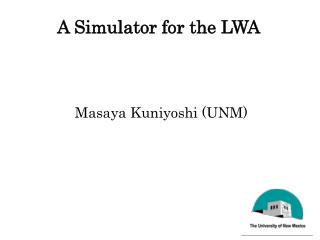# A Simulator for the LWA - PowerPoint PPT PresentationDownload PresentationA Simulator for the LWA

A Simulator for the LWADownload Presentation## A Simulator for the LWA

- - - - - - - - - - - - - - - - - - - - - - - - - - - E N D - - - - - - - - - - - - - - - - - - - - - - - - - - -
##### Presentation Transcript

1. A Simulator for the LWA Masaya Kuniyoshi (UNM)

2. Outline 1.Station Beam Model 2.Asymmetry Station Beam 3.Station Beam Error 4.Summary

3. (Aaron Cohen LWA Memo Series )

4. 256 dipoles (Leonid Kogan LWA Memo Series )

5. ( D・ u ) Ψ = j j λ 2π ( D・ S ) Φ = j j λ 。 。 = Zenith S (0,0 ） 。 。 S (10,0 ) Simulation model for a station beam 255 E(θ,φ)=ΣGaussian(θ,φ)exp(iP )exp(Ψ－Φ）＋Noise j j j j=0 Gaussian(θ,φ) = individual primary beam Station beam Station beam φ[degree] φ[degree] θ[degree] θ[degree]

6. Normalized Power Pattern θ[°] (angle form zenith)

7. Normalized Power Pattern θ[°] (angle form zenith)

8. Normalized Power Pattern θ[°] (angle form zenith)

9. Normalized Power Pattern θ[°] (angle form zenith)

10. Normalized Power Pattern θ[°] (angle form zenith)

11. Normalized Power Pattern θ[°] (angle form zenith)

12. Normalized Power Pattern θ[°] (angle form zenith)

13. Normalized Power Pattern θ[°] (angle form zenith)

14. Normalized Power Pattern θ[°] (angle form zenith)

15. Normalized Power Pattern θ[°] (angle form zenith)

16. Normalized Power Pattern θ[°] (angle form zenith)

17. Normalized Power Pattern θ[°] (angle form zenith)

18. Normalized Power Pattern θ[°] (angle form zenith)

19. Normalized Power Pattern θ[°] (angle form zenith)

20. Normalized Power Pattern θ[°] (angle form zenith)

21. Normalized Power Pattern θ[°] (angle form zenith)

22. Normalized Power Pattern θ[°] (angle form zenith)

23. Normalized Power Pattern θ[°] (angle form zenith)

24. Normalized Power Pattern θ[°] (angle form zenith)

25. Normalized Power Pattern θ[°] (angle form zenith)

26. Normalized Power Pattern θ[°] (angle form zenith)

27. Normalized Power Pattern θ[°] (angle form zenith)

28. Symmetry Normalized Power Pattern θ[°] (angle form zenith)

29. Asymmetry Normalized Power Pattern θ[°] (angle form zenith)

30. Asymmetry Normalized Power Pattern θ[°] (angle form zenith)

31. Normalized Power Pattern ８° ９° １３° ２８° θ[°] (angle form zenith)

32. 80MHz 20MHz 50MHz Asymmetry rate HPBW left side/ HPBW right side θ[°] angle from zenith

33. θ Dsinθ D As the angle θgoes from 0 to π/2, the value of cosθ(differentiation of sinθ) gets smaller. As a result, the beam becomes asymmetric. This effect increases as the frequency decreases.

34. θ ＝-70° Zenith = 0 ° Beam pattern peak θ (degree)

35. θ ＝-60 ° Zenith = 0 ° Beam pattern peak θ (degree)

36. θ ＝-50 ° Zenith = 0 ° Beam pattern θ (degree)

37. θ ＝-40 ° Zenith = 0 ° Beam pattern θ (degree)

38. θ ＝-30 ° Zenith = 0 ° Beam pattern θ (degree)

39. θ ＝-20 ° Zenith = 0 ° Beam pattern θ θ (degree)

40. θ ＝-10 ° Zenith = 0 ° Beam pattern θ θ (degree)

41. θ ＝0 ° Zenith = 0 ° Beam pattern θ θ (degree)

42. θ ＝10 ° Zenith = 0 ° Beam pattern θ θ (degree)

43. θ ＝20 ° Zenith = 0 ° Beam pattern θ θ (degree)

44. θ ＝30 ° Zenith = 0 ° Beam pattern θ θ (degree)

45. θ ＝40 ° Zenith = 0 ° θ

46. θ ＝50 ° Zenith = 0 ° Beam pattern θ θ (degree)

47. θ ＝60 ° Zenith = 0 ° Beam pattern peak θ θ (degree)

48. θ ＝70 ° Zenith = 0 ° Beam pattern peak θ θ (degree)

49. θ ＝-70 ° Grating lobe Zenith = 0 ° λ ・57.3≒43° d Beam pattern Grating lobe θ (degree)

50. θ ＝-60 ° Grating lobe Zenith = 0 ° Beam pattern Grating lobe θ (degree)